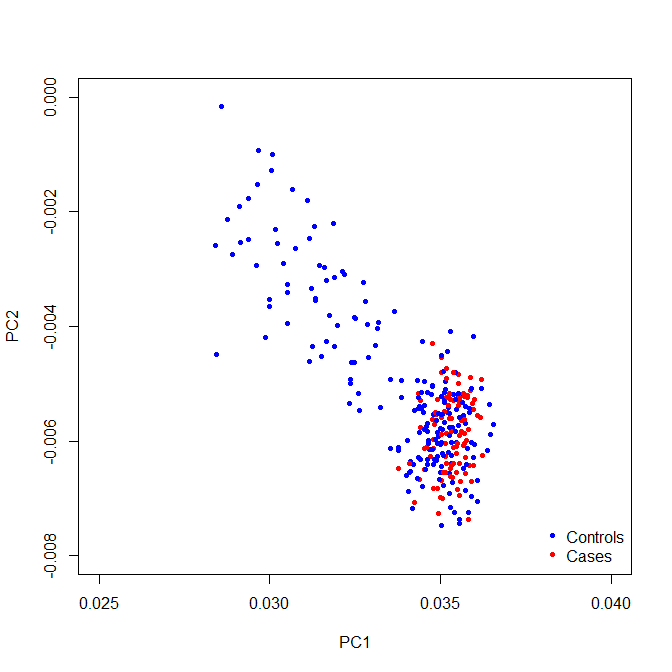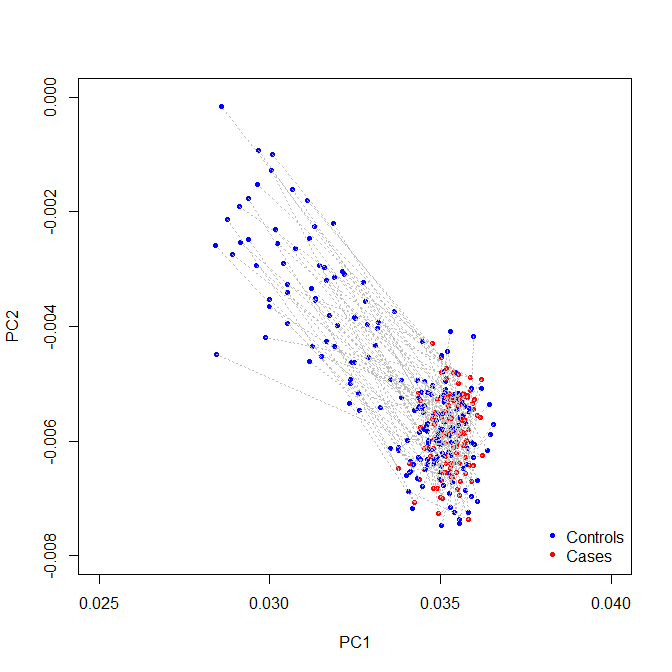# PCAmatchR: Match Cases to Controls Based on Genotype Principal Components

## Description

PCAmatchR matches cases to controls based on genotype principal components (PC). In order to produce more genetically similar matches, a weighted Mahalanobis distance metric (Kidd et al. (1987)) is used to determine matches. Weights are equal to the percent variance explained by each PC.

## Installation

The release version of PCAmatchR can be installed from CRAN:

install.packages("PCAmatchR")

The development version of the PCAmatchR package can be installed from the GitHub repository by using the devtools package:

devtools::install_github("machiela-lab/PCAmatchR")

PCAmatchR depends on the optmatch package which must be manually installed from CRAN:

install.packages("optmatch")

Following installation, attach the PCAmatchR and optmatch packages with:

library(PCAmatchR)
library(optmatch)
setMaxProblemSize(size = Inf) # optmatch option to allow for large matching problems. See optmatch documentation for full description. 

## Usage

Here we perform a hypothetical example of case-control matching using the Phase 3 data release of 1000 Genomes Project, which contains genotype data from 2,504 individuals from 26 distinct populations (available at https://www.cog-genomics.org/plink/2.0/resources).

### Available Data

Within PCAmatchR, we include sample data containing information about population, gender, and the first 20 principal components and eigenvalues calculated from 2,504 individuals in the Phase 3 data release of the 1000 Genomes Project. The example principal component analysis was conducted with PLINK using a set of ancestry informative SNPs (Yu et al. (2008)). The data files are contained within:

• PCs_1000G, A sample dataset containing information about population, gender, and the first 20 principal components calculated from 2,504 individuals in the Phase 3 data release of the 1000 Genomes Project.

• eigenvalues_1000G, A sample dataset containing the first 20 eigenvalues calculated from 2,504 individuals in the Phase 3 data release of the 1000 Genomes Project.

• eigenvalues_all_1000G, A sample dataset containing all eigenvalues calculated from 2,504 individuals in the Phase 3 data release of the 1000 Genomes Project.

# Load required packages

# Create PC data frame
pcs<- as.data.frame(PCs_1000G[,c(1,5:24)])

# Create eigenvalues vector
eigen_vals<- c(eigenvalues_1000G)$eigen_values # Create full eigenvalues vector all_eigen_vals<- c(eigenvalues_all_1000G)$eigen_values

### Case and Control Populations

For this example analysis, we select individuals from the ESN (Esan in Nigeria) population as cases (N=99), while all remaining samples are used as the control population (N=2,405):

covariate_data<- as.data.frame(PCs_1000G[,1:4])
covariate_data$case <- ifelse(covariate_data$pop=="ESN", c(1), c(0))

### Case-Control Matching

Matching is performed using match_maker. Within this example, cases and controls are 1:2 matched on the first 20 PCs:

match_maker_output<- match_maker(PC = pcs,
eigen_value = eigen_vals,
data = covariate_data,
ids = c("sample"),
case_control="case",
num_controls = 2,
eigen_sum = sum(all_eigen_vals))

Derived matches are contained within the matches object. The weighted Mahalanobis distance metric between case and control pairs is detailed within the match_distance variable:

PCA_matches<- match_maker_output$matches PCA_matches$match_distance

### Note

The all_eigen_vals file is not needed to run match_maker. The user can directly supply the scalar sum of all eigen values to the match_maker function through the eigen_sum input. In the above example, the sum of all the eigenvalues was 2722.856. This value is used to weight each eigen value to determine the percentage of variance explained.

If using PLINK to perform the PCA (e.g., --pca flag), there are multiple options to calculate the sum of all eigen values instead of having to generate all PCs:

• The first is to create a relationship matrix using --make-rel in PLINK. The diagonal of this matrix is equal to the eigen values. Summing the diagonal will obtain the integer to input into eigen_sum. This approach may not be feasible when working with a large number of cases and controls, due to the file size.
• The second is to generate the GCTA inbreeding coefficient report using --ibc in PLINK. This will output a file with 6 columns. Column 4 (Fhat1), gives the variance-standardized relationship minus 1. Add 1 to each entry in this column, and then sum all entries. This will result in the total sum of the eigen values (i.e., the total variance explained). This value can then be used as the input to eigen_sum.

### Case-Control Matching Visualization

The distance between matches can be visualized using plot_maker:

plot_maker(data=match_maker_output,
x_var="PC1",
y_var="PC2",
case_control="case",
line=F,
xlim = c(0.025,0.04),
ylim = c(-0.008,0.00))The function further allows for connections between matches:

plot_maker(data=match_maker_output,
x_var="PC1",
y_var="PC2",
case_control="case",
line=T,
xlim = c(0.025,0.04),
ylim = c(-0.008,0.00))